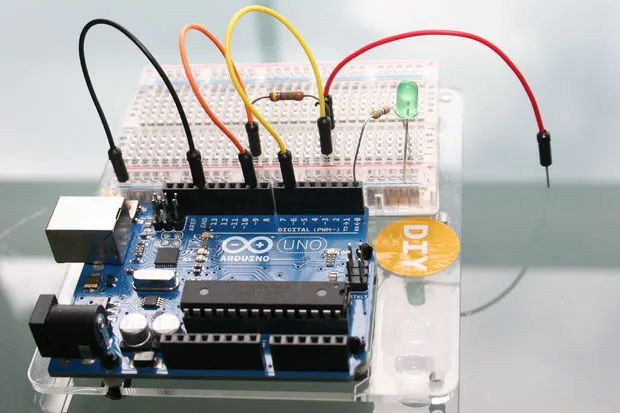# Just Veggin with an Arduino Beetbox

Bring Touch Control to the Arduino. Use interesting touch sensors like Carrots or Beets to make a "Beetbox"

Full instructions provided1,732## Things used in this project

### Hardware componentsArduino UNO & Genuino UNO
×1
 Root Vegetables such as a Beet
×3LED (generic)
×1
×1
 560 Ohm resistor
×1
 4.7M Ohm resistor or a value similar to that, one for each sense channel
×3
 WaveHC library for Arduino
×1
×1
 Wires
×1

## Code

### file_6768.txt

C/C++
```void setup()
{
....some setup stuff....
}

void loop()
{
total1 =  cs_9_6.capacitiveSensor(30);        // Measure the Cap Sense Value from the Sensor

if (total1 > THRESHOLD) {
digitalWrite(LedPin, HIGH);
}
else {
digitalWrite(LedPin,LOW);
}
}
```

### file_6766.txt

C/C++
```void loop()
{
total1 =  cs_9_6.capacitiveSensor(30);        // Measure the Cap Sense Value from the Sensor

// Touch the Sensor while the LED is HIGH to Calibrate the TOUCH value
if (i<50) {                                      // Calibrate Sensor baseline to Start
digitalWrite(LedPin, HIGH);
calVal1= 0.1*float(total1) + 0.9*calVal1;
Serial.println(calVal1);                // print calibartion value during calibration cycle
delay(50);
digitalWrite(LedPin, LOW);
delay(50);
i++;
}
}
```

### file_6769.txt

C/C++
```#include <CapacitiveSensor.h>

/*
* Uses a high value resistor e.g. 10M between send pin and receive pin
* Resistor effects sensitivity, experiment with values, 50K - 50M. Larger resistor values yield larger sensor values.
* Receive pin is the sensor pin.
*/

#define error(msg) error_P(PSTR(msg))

CapacitiveSensor   cs_9_6 = CapacitiveSensor(9,6);        // 10M resistor between pins 9 & 6, pin 6 is sensor pin, add a wire and or foil if desired

float calVal1 = 0.0;

float total1 = 0.0;

int i = 0;
int LedPin = 2;            // Pin the LED is connected to

void setup()
{
Serial.begin(9600);

cs_9_6.reset_CS_AutoCal();  // autocalibrate channel 1
cs_9_6.set_CS_AutocaL_Millis(0x00001000);     // autocalibrate interval on channel 1

pinMode(LedPin, OUTPUT);
i = 0;                  // Initialise some values
calVal1 = 0.0;
}

void loop()
{
total1 =  cs_9_6.capacitiveSensor(30);        // Measure the Cap Sense Value from the Sensor

// Touch the Sensor while the LED is HIGH to Calibrate the TOUCH value
if (i<50) {                                      // Calibrate Sensor baseline to Start
digitalWrite(LedPin, HIGH);
calVal1= 0.1*float(total1) + 0.9*calVal1;
Serial.println(calVal1);                // print calibration value during calibration cycle
delay(50);
digitalWrite(LedPin, LOW);
delay(50);
i++;
}
else {                                      // Calibration is Over
Serial.print(calVal1);
Serial.print("\t");
Serial.println(total1);                  // print sensor output 1

if (total1 > 0.8*calVal1) {
digitalWrite(LedPin, HIGH);
}
else {
digitalWrite(LedPin,LOW);
}
}
}
```

### file_6763.txt

C/C++
```#include <CapacitiveSensor.h>
#include <WaveHC.h>
#include <WaveUtil.h>

/*
* Uses a high value resistor e.g. 10M between send pin and receive pin
* Resistor effects sensitivity, experiment with values, 50K - 50M. Larger resistor values yield larger sensor values.
* Receive pin is the sensor pin.
*/

SdReader card;    // This object holds the information for the card
FatVolume vol;    // This holds the information for the partition on the card
FatReader root;   // This holds the information for the volumes root directory
FatReader file;   // This object represent the WAV file for a pi digit or period
WaveHC wave;      // This is the only wave (audio) object, since we will only play one at a time

char filename;

// Uncomment next line to enable Debug Serial.print() statements
//#define DEBUG

#define error(msg) error_P(PSTR(msg))

CapacitiveSensor   cs_9_6 = CapacitiveSensor(9,6);        // 10M resistor between pins 9 & 6, pin 6 is sensor pin, add a wire and or foil if desired
CapacitiveSensor   cs_9_7 = CapacitiveSensor(9,7);
CapacitiveSensor   cs_9_8 = CapacitiveSensor(9,8);

float calVal1 = 0.0;
float calVal2 = 0.0;
float calVal3 = 0.0;
float total1 = 0.0;
float total2 = 0.0;
float total3 = 0.0;
int i = 0;

void setup()
{
Serial.begin(9600);

if (!card.init()) {
error("Card init. failed!");
}
if (!vol.init(card)) {
error("No partition!");
}
if (!root.openRoot(vol)) {
error("Couldn't open dir");
}

PgmPrintln("Files found:");
root.ls();

cs_9_6.reset_CS_AutoCal();  // autocalibrate channel 1
cs_9_7.reset_CS_AutoCal();  // autocalibrate channel 2
cs_9_8.reset_CS_AutoCal();  // autocalibrate channel 3

cs_9_6.set_CS_AutocaL_Millis(0x00001000);     // autocalibrate interval on channel 1
cs_9_7.set_CS_AutocaL_Millis(0x00001000);     // autocalibrate interval on channel 2
cs_9_8.set_CS_AutocaL_Millis(0x00001000);     // autocalibrate interval on channel 3

pinMode(14, OUTPUT);
}

void loop()
{
total1 =  cs_9_6.capacitiveSensor(30);
total2 =  cs_9_7.capacitiveSensor(30);
total3 =  cs_9_8.capacitiveSensor(30);

if (i<60) {                                                  // Calibrate Sensor baseline
digitalWrite(14, HIGH);
calVal1= 0.1*float(total1) + 0.9*calVal1;
i++;
}
else if (i<120) {                                      // Calibrate Sensor baseline
digitalWrite(14, LOW);
calVal2= 0.1*float(total2) + 0.9*calVal2;
i++;
}
else if (i<180) {                                      // Calibrate Sensor baseline
digitalWrite(14, HIGH);
calVal3= 0.1*float(total3) + 0.9*calVal3;
i++;
}
else {
digitalWrite(14,LOW);
}

#ifdef DEBUG
Serial.print(total1);                  // print sensor output 1
Serial.print("\t");
Serial.print(total2);                // print sensor output 2
Serial.print("\t");
Serial.println(total3);                // print sensor output 3

Serial.print("CAL: \t");
Serial.print(calVal1);                  // print sensor output 1
Serial.print("\t");
Serial.print(calVal2);                // print sensor output 2
Serial.print("\t");
Serial.println(calVal3);                // print sensor output 3
#endif

if (total1 > 0.8*calVal1 && total2 > 0.8*calVal2) {
strcpy_P(filename, PSTR("BUFF1.WAV"));
Serial.println(filename);
playfile(filename);
}
else if (total2 > 0.8*calVal2 && total3 > 0.8*calVal3) {
strcpy_P(filename, PSTR("HAT.WAV"));
Serial.println(filename);
playfile(filename);
}
else if (total1 > 0.8*calVal1) {
strcpy_P(filename, PSTR("CLICK1.WAV"));
Serial.println(filename);
playfile(filename);
}
else if (total2 > 0.8*calVal2) {
strcpy_P(filename, PSTR("BOOM1.WAV"));
Serial.println(filename);
playfile(filename);
}
else if (total3 > 0.8*calVal3) {
strcpy_P(filename, PSTR("BOOMHAA1.WAV"));
Serial.println(filename);
playfile(filename);
}

//   delay(10);                             // arbitrary delay to limit data to serial port
}

/////////////////////////////////// HELPERS

/*
* print error message and halt
*/
void error_P(const char *str) {
PgmPrint("Error: ");
SerialPrint_P(str);
sdErrorCheck();
while(1);
}
/*
* print error message and halt if SD I/O error
*/
void sdErrorCheck(void) {
if (!card.errorCode()) return;
PgmPrint("\r\nSD I/O error: ");
Serial.print(card.errorCode(), HEX);
PgmPrint(", ");
Serial.println(card.errorData(), HEX);
while(1);
}
/*
* Play a file and wait for it to complete
*/
void playcomplete(char *name) {
playfile(name);
while (wave.isplaying);

// see if an error occurred while playing
sdErrorCheck();
}
/*
* Open and start playing a WAV file
*/
void playfile(char *name) {
if (wave.isplaying) {// already playing something, so stop it!
wave.stop(); // stop it
}
if (!file.open(root, name)) {
PgmPrint("Couldn't open file ");
Serial.print(name);
return;
}
if (!wave.create(file)) {
PgmPrintln("Not a valid WAV");
return;
}
// ok time to play!
wave.play();
}
```

## Credits

### Anthony Kelly

5 projects • 25 followers
Hobby Arduino user. Software Hacker. Ph.D. Electronic Engineering. Specialist in IC design, digital signal processing.